0%

# 开关电源补偿环路设计（1）：基础部分-以Buck 为例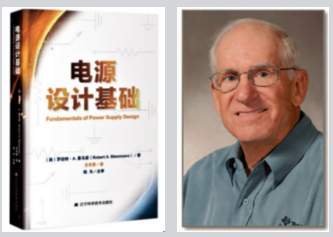## 一：buck变换器建模

buck变换器是最简单而经典的开关电源拓扑之一，其详细组成可见图1。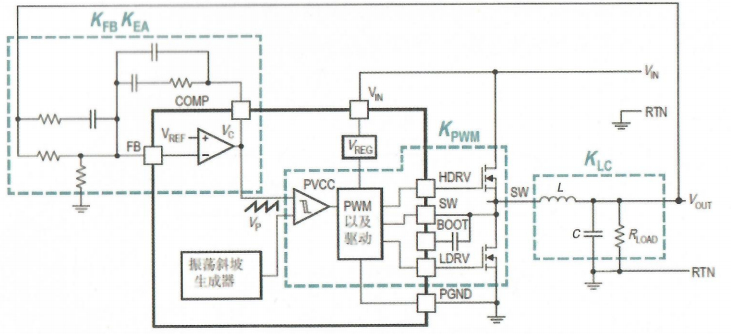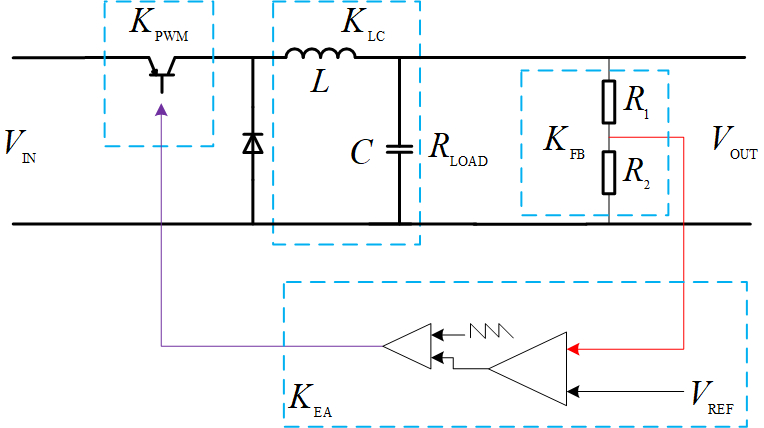​ 由图1可知buck变换器可分为多个模块，各个模块的定义见下表1

$K_{FB}$ 反馈电阻分压器，用于获取输出电压
$K_{EA}$ 带有频率补偿的误差放大器
$K_{PWM}$ 脉宽调制增益
$K_{LC}$ 输出滤波器增益

​ 其实$K_{FB}$是一个直流缩放因子（电阻分压器），如果必需考虑其非理想等效模式（LRC模型）则将其作为$K_{EA}$的一部分。
​ 如图2所示为整个稳压调节器的反馈框图。### 1.1 反馈网络增益$K_{FB}$

​ 反馈网络增益$K_{FB}$模块决定了参考电压和输出电压之间的比例关系。通常来说，我们希望这种关系是一个固定的值。因此，通常只用一个电阻分压器来实现，我们唯一关心的是它的 比例DC值。
​ 无论$V_{OUT}$如何变化，迫使反馈点（求和点）维持在参考值。在反馈点没有电压变化的情况下，通过分压器下端电阻的电流无变化，站在频率的角度其有效数值为无限大。而在分压器中，最重要的是上端电阻，因为除了输出电压检测之外，它的阻抗将成为补偿网络的一部分。

### 1.2 脉宽调制增益$K_{PWM}$

​ 将PWM级定义为电路的一部分，基于误差放大器$V_{C}$的模拟输出电压调节开关管的占空比$D$，功率开关管在节点$SW$处开关启闭。其工作波形如图3所示，其占空比为$\frac{V_{C}}{V_{P}}$。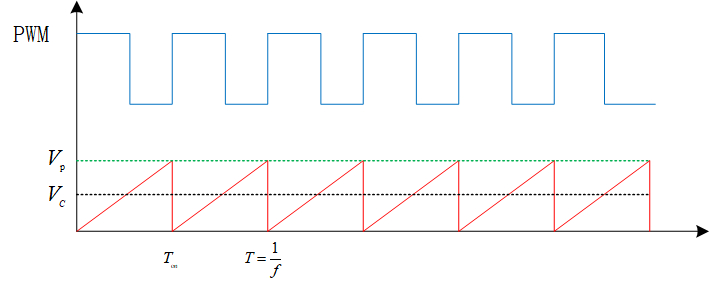### 1.3 输出滤波器增益$K_{LC}(s)$

​ 输出滤波器的作用是将开关管波形转换为输出端的直流电压$V_{OUT}$，本质上来说这是一个分压网络，由电感$L_{s}$、电容$\frac {1}{C_{s}}$以及负载阻抗$R_{s}$并联组成，如图4所示。本质上是一个串联电感和并联电容的“输出滤波+分压”。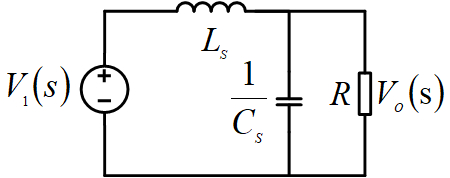​ 其中$p_{1}$和$p_{2}$是这个二阶系统的两个极点，它们是通过将增益方程的分母设置为零并求解根得到（分子的根将成为零点，但是这个方程中没有零点，接下来会谈到更多的关于零极点的内容）。
​ 通过这个方程的数值解，我们将会得到增益的幅度和相位，它们都是频率的函数。因为极点和零点以特定的方式起作用，可以通过图形化方法来获得足够的信息，即相对于对数频率坐标，根据增益（dB增益对数的20倍）和相位（度）作图，这称之为波特图，它可以很容易地可视化显示出系统稳定特性。
​ 求解上面的传递函数方程，j进而可以绘制出增益曲线，它是频率的函数，可知，L-C滤波器即存两个极点，而且已经知道如下情况∶

$f\ll \frac{1}{2\pi }\sqrt{\frac{1}{LC} }$ 增益=1，相位=0
$f= \frac{1}{2\pi }\sqrt{\frac{1}{LC} }$ 增益和相位正处于过渡状态，在这个频率点$f_{DP}$,增益为$R\sqrt{\frac{1}{LC} }$，相位=$90^{o}$
$f\gg \frac{1}{2\pi }\sqrt{\frac{1}{LC} }$ 增益为$-\frac{1}{w^{2}LC}$，相位=$180^{o}$。注意到增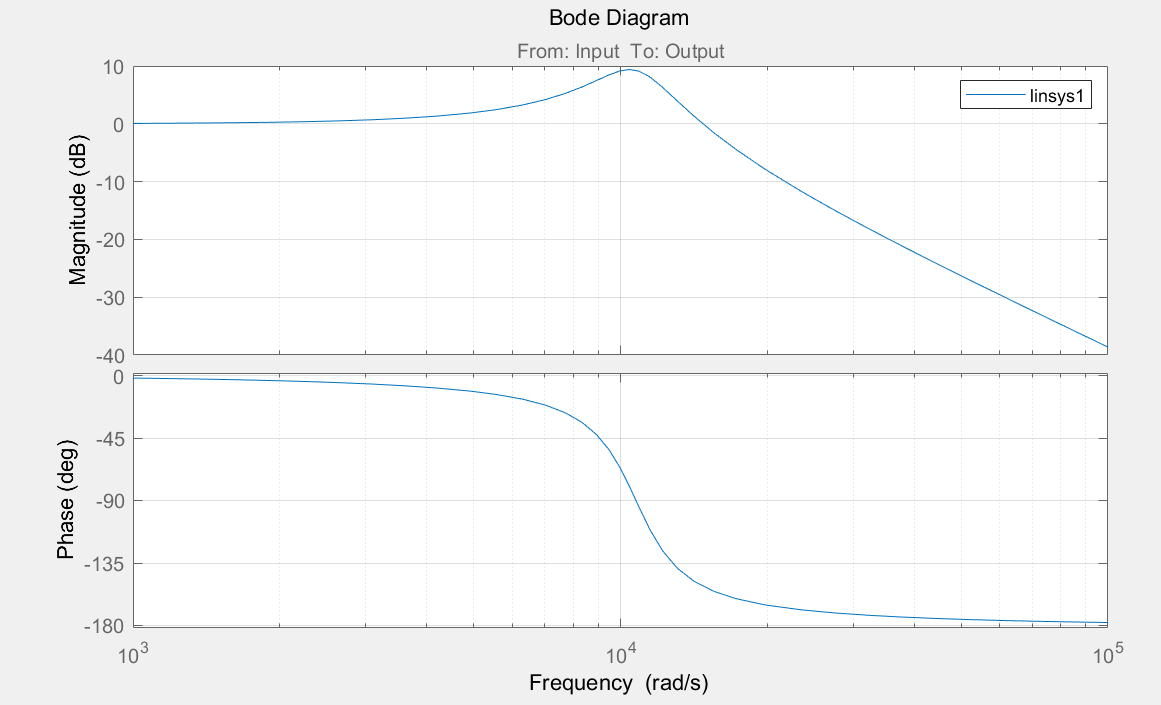### 1.4 误差放大器增益$K_{EA}$

​ 由之前的系统定义可知，输出检测信号接在负反馈的反相端，所以系统具有$180^{o}$的相移，而输出滤波器中可能出现另一个180°的相移。因此，该系统在频率高于输出滤波器截止频率的情况下，必然是不稳定的。

​ 因此，误差放大器必须为精确电压调节提供高增益的同时还要确保在满足动态响应要求的整个工作频率范围内，总相移保持小于$360^{o}$。这可以通过一个运算放大器（OPA）实现，见图6。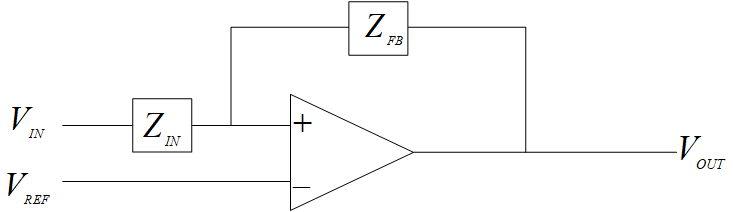​ 图 6中，放大器的总增益$K_{EA}$将为$\frac{Z_{FB}}{Z_{IN}}$，当$K_{EA}=\frac{Z_{FB}}{Z_{IN}}$为直流情况时，有$180^{o}$的相位偏移。这些阻抗是与频率相关的，它们的任何变化将导致增益和相位发生变化。因为降低$Z_{IN}$的阻抗将会增加总增益，而降低$Z_{FB}$阻抗将降低总增益。通常来说，我们通过电阻和电容的串并联组合来改变阻抗。更具体地说，利用电容的频率特性，R和C的并联网络，在低频时具有以R值开始的阻抗，即以电阻特性为主。然后，在截止频率$f_{C}$处，电容的阻抗开始作用，随着频率的进一步增加，并联阻抗以$-20dB/$十倍频程（$-6dB/$倍频程）的斜率下降。在相同的频率范围内，相移从低频处的$0^{o}$变为在截止频率处的$-90^{o}$。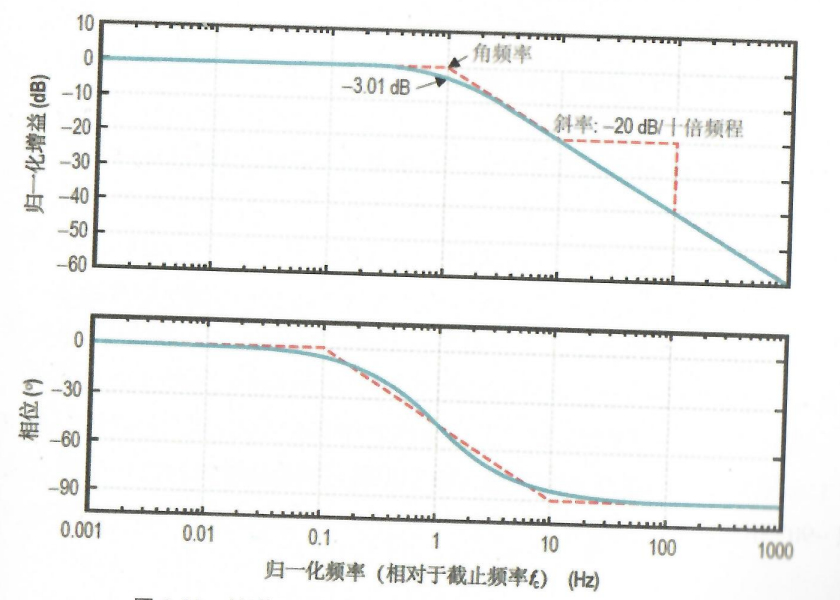​ 在图7的波特图上可以很容易地看到这些特性。电阻和电容的串联网络阻抗具有类似但相反的特性。它在直流时阻抗为无穷大，随着频率增加以$-20dB/$十倍频程（$-6dB/$倍频程）的斜率下降。直到达到截止频率$f_{c}$，在此之后的频段上，阻抗变得平坦并固定为值$R$。相位也具有与$R-C$串联网络相反的特性，从低频开始于$-90^{o}$。并且在剧过截止顺率后上升到$0^{o}$。
​ 使用波特图来绘制增益（用dB表达）和相位（相对于频率的对数）曲线的优点是很明显的。虽然理论实际曲线是连续的，但可以用直线来逼近它们，其中增益斜率的变化正好在$f_{c}$处出现，而相移开始于大约$0.1f_{c}$处，在$f_{c}$处为45°，并且在$10×f_{c}$处结束。

​ 另外，截止频率$f_{c}$出现在$R$和$C$的阻抗相等的地方，即为$f_{c}= \frac{1}{2πRC}$。上图中的实线和虚线分别为实际曲线和直线逼近线，在绝大多数分析中，因为这个误差足够小，所以这种近似方法是可行的。
​ 如果将这些$R-C$组合应用到运算放大器中，有许多不同的组合，它们对增益和相位的改变效果是不同的∶

$Z_{IN}$为$R-C$串联 这会增加一个极点（产生-90°相移），并且增益减少
$Z_{IN}$为$R-C$并联 这会增加一个零点（产生+90°相移），并且增益增加
$Z_{FB}$为$R-C$串联 这会增加一个零点（产生+90°相移），并且增益减少
$Z_{FB}$为$R-C$并联 这会增加一个极点（产生-90°相移），并且增益减少

### 1.5 稳定性的相关考虑

1 已经不用再考虑$K_{FB}$的作用，因为这一项只影响系统直流工作点
2 总增益将与直流占空比无关，但与直流输入电压相关
3 分母中存在R，如果R=0即空载时表示系统会不稳定，但实际上电路，将首先进入DCM工作。在这种情况下，这个二阶系统本质上（退化为）是一阶的，所以这时小信号分析不再适用
4 我们已经将$H(s) \times G(s)$假定简化为正数，但负反馈隐含地给出相位变化为 180°，因此，在任何增益大于1的情况下，系统可能出现不稳定

### 1.6 误差放大器的补偿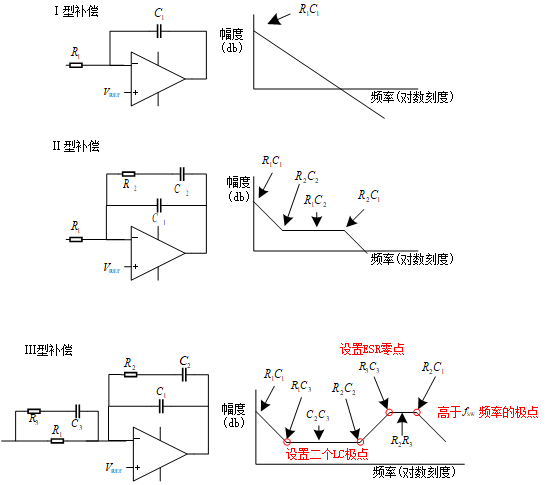​ 图8最常见的三种补偿电路，以及简化的增益特性（直线逼近曲线）
​ 上述增益曲线图都是由补偿器中的零极点决定的，它们的值（决定了特征频率的位置）是相对于输出滤波器电感和电容的值而言的（包括输出电容的ESR)。

#### 1.6.1Ⅰ型补偿

I型的补偿无疑是最简单的，利用一个单极点，将总增益降低到1以下，其频率远低于任何其他电路元件影响的频率。具体而言，设定频率$\frac{1}{2πR_{1}C_{1}}$，使得在小于$0.1f_{DP}$频率（输出滤波器截止频率的$\frac{1}{10}$）时，总增益会小于0dB。

#### 1.6.2 Ⅱ型补偿

​ 这个补偿电路在低频下和Ⅰ型补偿一样，在低频时同样以单极点开始，但是在环路增益仍为正值的情况下，首先通过引入一个零点来消除输出滤波器的其中一个极点，然后在较高的频率再引入一个极点将增益降低到0dB以下
​ 通常，这仍不足以稳定大多数电压模式控制的系统，因为在具有正增益的情况下，初始极点和剩余的输出滤波器极点相位总和将达到180°，这会导致系统

#### 1.6.3 Ⅲ型补偿

​ Ⅲ型补偿，相对于前二者补偿来说显然要复杂得多，但是通过在两个滤波器极点频率处或附近引入两个零点，可以解决输出滤波器双极点问题。利用具有增益上升和+90°相移的零点来抵消具有增益下降和-90°相移的极点。
​ 我们将这些零点放置在需要相位提升的地方，使得有用的增益显著扩展到超过输出滤波器的滚降点，但是仍然要在更高的频率处再增加两个极点使增益最终减至小于单位增益。需要注意的是，当一对零点（或极点）的位置是很接近的时候，由补偿网络的电容，而不是电阻来决定它们之间的增益。